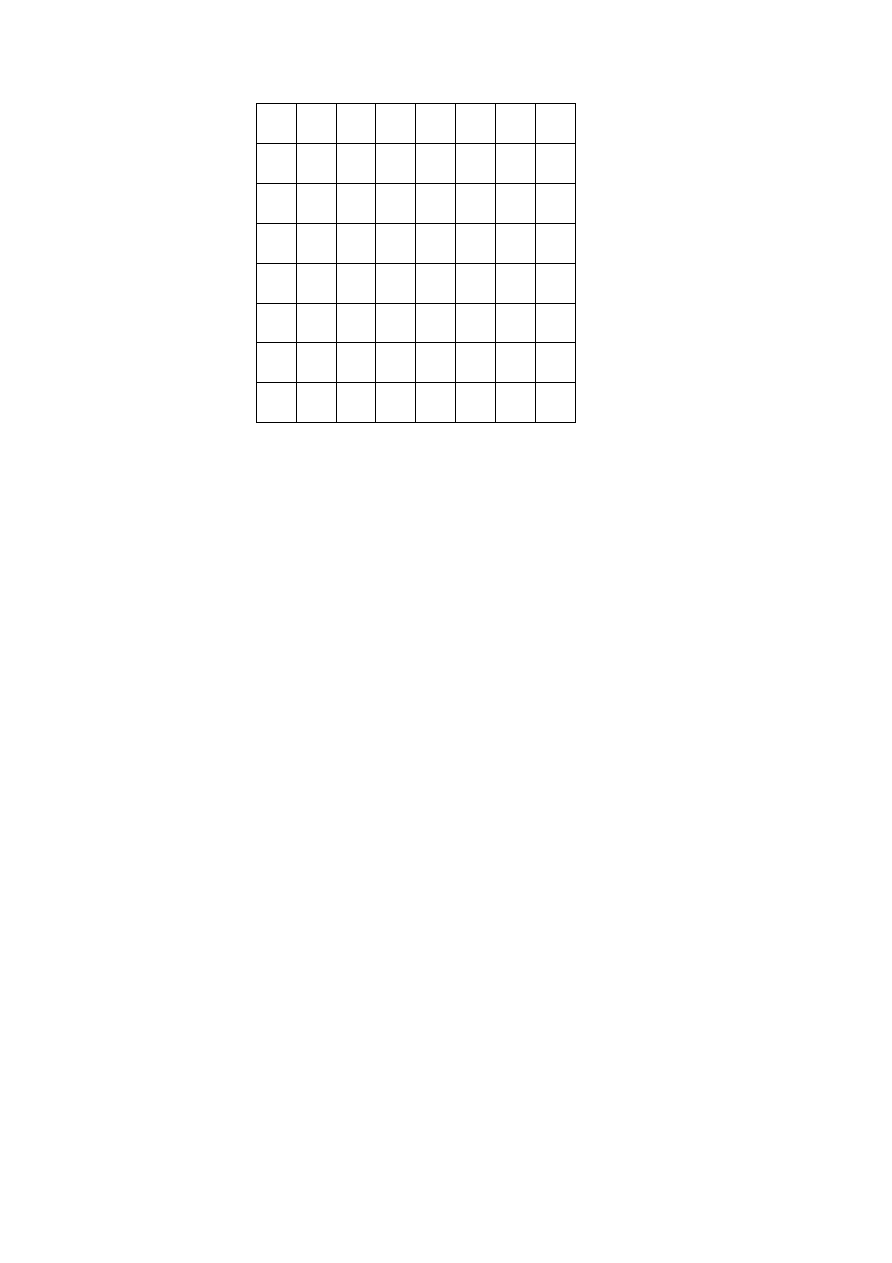ISO/IEC 10918-1 : 1993(E)
1
0
1
1
1
5
1
6
14
15
27
28
1
2
1
4
1
7
13
16
26
29
42
1
3
1
8
12
17
25
30
41
43
1
9
11
18
24
31
40
44
53
10
19
23
32
39
45
52
54
20
22
33
38
46
51
55
60
21
34
37
47
50
56
59
61
35
36
48
49
57
58
62
63
Figure A.6 ­ Zig-zag sequence of quantized DCT coefficients
A.5
Arithmetic procedures in lossless and hierarchical modes of operation
In the lossless mode of operation predictions are calculated with full precision and without clamping of either overflow or
underflow beyond the range of values allowed by the precision of the input. However, the division by two which is part of
some of the prediction calculations shall be approximated by an arithmetic-shift-right by one bit.
The two's complement differences which are coded in either the lossless mode of operation or the differential frame
coding in the hierarchical mode of operation are calculated modulo 65 536, thereby restricting the precision of these
differences to a maximum of 16 bits. The modulo values are calculated by performing the logical AND operation of the
two's complement difference with X'FFFF'. For purposes of coding, the result is still interpreted as a 16 bit two's
complement difference. Modulo 65 536 arithmetic is also used in the decoder in calculating the output from the sum of
the prediction and this two's complement difference.
30
CCITT Rec. T.81 (1992 E)